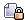###Author Topic: 5 Law of (Co)Sines problems  (Read 1068 times)

0 Members and 1 Guest are viewing this topic.

#### trust

• Guest##### 5 Law of (Co)Sines problems
« on: May 29, 2006, 09:07:26 PM »
1. Solve:
A=?, a=3, B=?, b=4, C=53°, c=?

2. Use law of sines to solve for all possible triangles that satisfy A=43.1°, a=186.2, b=248.6

Currently I have: C=206.96, B=6.32, and c=130.58

3.Sketch/Solve:

Two ships leave port at the same time. One travels 20 mi/hr N32°E and the other 28mi/hr S42°E. How far apart are they after 2 hours?

For these two I included my sketches of the given info:

4. Sketch/Solve:

A satellite orbiting the earth passes directly overhead at observations stations in Phoenix and Los Angeles, 340 miles apart. At an instant when the satellite is between these 2 stations, its angle of elevation is simultaneously observed to be 60° at Phoenix and 75° at LA. How far is the satellite from LA?

so far I have: A=60, a = 416.41, B=75, b=464.45, C=45, c=340

5. Sketch/Solve:

A pilot is flying over a straight highway. He determines the angles of dpression to two mileposts (A and B), 5 miles apart, to be 32° and 48° respectively. Find the distance of the plane from milepost A, and the elevation of the plane. (Assume plane is inbetween mileposts.)

so far I have: A=32, B=48, C=100, c=5. Then I extended the left side of triangle ABC, to create ACD, with A having a 58 degree angle, C having a 32 degree angle, and D having a 90 degree angle.

Thanks.

Edit: Nevermind
« Last Edit: June 02, 2006, 09:54:15 AM by OG Trust »